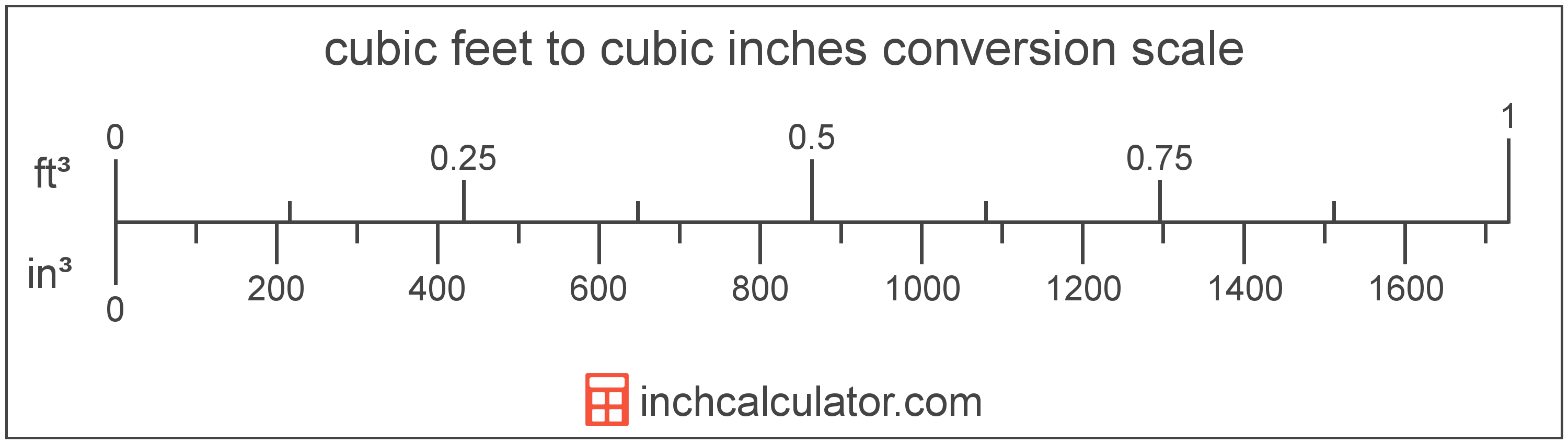# Cubic Feet to Cubic Inches Converter

Enter the volume in cubic feet below to get the value converted to cubic inches.

(find cubic feet)
Results in Cubic Inches:1 ft³ = 1,728 in³

Do you want to convert cubic inches to cubic feet?

## How to Convert Cubic Feet to Cubic Inches

To convert a measurement in cubic feet to a measurement in cubic inches, multiply the volume by the following conversion ratio: 1,728 cubic inches/cubic foot.

Since one cubic foot is equal to 1,728 cubic inches, you can use this simple formula to convert:

cubic inches = cubic feet × 1,728

The volume in cubic inches is equal to the volume in cubic feet multiplied by 1,728.

For example, here's how to convert 5 cubic feet to cubic inches using the formula above.
cubic inches = (5 ft³ × 1,728) = 8,640 in³### How Many Cubic Inches Are in a Cubic Foot?

There are 1,728 cubic inches in a cubic foot, which is why we use this value in the formula above.

1 ft³ = 1,728 in³

## What Is a Cubic Foot?

The cubic foot is a unit of volume that is equal to the space consumed by a cube having sides one foot on each edge.

The cubic foot is a US customary and imperial unit of volume. A cubic foot is sometimes also referred to as a cubic ft. Cubic feet can be abbreviated as ft³, and are also sometimes abbreviated as cu foot, cu ft, or CF. For example, 1 cubic foot can be written as 1 ft³, 1 cu foot, 1 cu ft, or 1 CF.

You can calculate volume using a cubic footage calculator if you have the dimensions of a space or object.

## What Is a Cubic Inch?

A cubic inch is a unit of volume equal to the space consumed by a cube with sides that are one inch in all directions. One cubic inch is equivalent to about 16.387 cubic centimeters or 0.554 fluid ounces.

The cubic inch is a US customary and imperial unit of volume. A cubic inch is sometimes also referred to as a cubic in. Cubic inches can be abbreviated as in³, and are also sometimes abbreviated as cu inch, cu in, or CI. For example, 1 cubic inch can be written as 1 in³, 1 cu inch, 1 cu in, or 1 CI.

## Cubic Foot to Cubic Inch Conversion Table

Table showing various cubic foot measurements converted to cubic inches.
Cubic Feet Cubic Inches
0.001 ft³ 1.728 in³
0.002 ft³ 3.456 in³
0.003 ft³ 5.184 in³
0.004 ft³ 6.912 in³
0.005 ft³ 8.64 in³
0.006 ft³ 10.37 in³
0.007 ft³ 12.1 in³
0.008 ft³ 13.82 in³
0.009 ft³ 15.55 in³
0.01 ft³ 17.28 in³
0.02 ft³ 34.56 in³
0.03 ft³ 51.84 in³
0.04 ft³ 69.12 in³
0.05 ft³ 86.4 in³
0.06 ft³ 103.68 in³
0.07 ft³ 120.96 in³
0.08 ft³ 138.24 in³
0.09 ft³ 155.52 in³
0.1 ft³ 172.8 in³
0.2 ft³ 345.6 in³
0.3 ft³ 518.4 in³
0.4 ft³ 691.2 in³
0.5 ft³ 864 in³
0.6 ft³ 1,037 in³
0.7 ft³ 1,210 in³
0.8 ft³ 1,382 in³
0.9 ft³ 1,555 in³
1 ft³ 1,728 in³

## References

1. National Institute of Standards and Technology, Specifications, Tolerances, and Other Technical Requirements for Weighing and Measuring Devices, Handbook 44 - 2019 Edition, https://nvlpubs.nist.gov/nistpubs/hb/2019/NIST.HB.44-2019.pdf